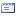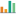### Students’ Mathematical Representation in Geometry Problem Solving Based Sex Differences

Adnan S(1), Dwi Juniati(2), Raden Sulaiman(3),
(1) Surabaya State University, Indonesia
(2) Department of Mathematics Education, Surabaya State University, Surabaya, Indonesia
(3) Department of Mathematics Education, Surabaya State University, Surabaya, IndonesiaCorresponding Author

#### Abstract

This study aims to describe the mathematical representation of students solving geometric problems based on sex differences. Both subjects have equivalent mathematical abilities based on the results of the math ability test. The results showed that the subject used mathematical representation in expressing his idea to solve geometry problems by using Polya's problem solving steps: (a) understanding the problem (b) devising the plan (c) carrying out the plan, and (d) looking back. The mathematical representations of female subjects in solving geometric problems are: understanding information and what is asked verbally and symbolically, carrying the plans visually in the form of geometric formulas and mathematics, carrying out planning by drawing, and manipulating mathematical models, at the stage of looking back the subject performed symbolic recalculation. While mathematical representations of male subjects in express their ideas to solve geometric problems by: understanding information and what is asked verbally, devising the plans in visual form in the form of images and then making mathematical formulas, carrying out the plans by manipulating mathematical models that has been made and looking back by doing recalculation and writing conclusions.

#### Keywords

Mathematical representation; Geometry problem solving; Sex difference;

#### Article DOI

DOI: https://doi.org/10.33122/ijtmer.v2i4.94

#### Article MetricsAbstract views : 231PDF views : 164

Pages: 184-187

PDF

### Refbacks

• There are currently no refbacks.